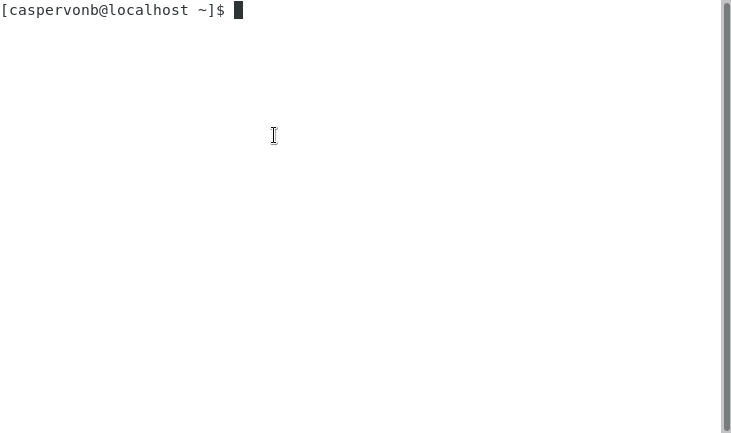# 鲜为人知的 JavaScript 陷阱## 箭头函数和对象字面量

const numbers = [1, 2, 3, 4];numbers.map(function(n) {return n * n;});

const numbers = [1, 2, 3, 4];numbers.map(n => n * n);

const numbers = [1, 2, 3, 4];numbers.map(n => { value: n });

const numbers = [1, 2, 3, 4];numbers.map(function(n) {value:nreturn;});

const numbers = [1, 2, 3, 4];numbers.map(n => ({ value: n }));

## 箭头函数和绑定

let calculator = {  value: 0,  add: (values) => {    this.value = values.reduce((a, v) => a + v, this.value);  },};calculator.add([1, 2, 3]);console.log(calculator.value);

let calculator = {  value: 0,  add: (values) => {    this.value = values.reduce((a, v) => a + v, this.value);  },};calculator.add([1, 2, 3]);console.log(calculator.value);

const adder = {  add: (values) => {    this.value = values.reduce((a, v) => a + v, this.value);  },};let calculator = {  value: 0};adder.add.call(calculator, [1, 2, 3]);

## 自动分号插入

return{  value: 42}

return;{  value: 42};

## 浅集合

let set = new Set();set.add([1, 2, 3]);set.add([1, 2, 3]);console.log(set.length);

let set = new Set();set.add([1, 2, 3].join(','));set.add([1, 2, 3].join(','));console.log(set.size);

## 类与暂时死区

let segment = new Segment();function Segment() {  this.x = 0;  this.y = 0;}

let segment = new Segment();class Segment {  constructor() {    this.x = 0;    this.y = 0;  }}

## Finally

Finally 是一个特殊情况。看一下以下代码片段：

try {  return true;} finally {  return false;}

## 小结

JavaScript 很容易入门，但很难精通。换句话说，开发人员需要搞清楚自己正在做什么，明白为什么要这样做，否则就很容易出错。

ECMAScript 6 及其含糖功能尤其如此。特别是箭头函数哪里都会冒出来。让我来猜的话，那是因为开发人员认为它们比常规函数更漂亮。但它们不是常规函数，因此无法替代后者。

AST Explorer 之类的工具还可以帮助你了解某些极端场景下的状况。人类和计算机往往会以不同的方式来解析事物。## 评论2019 年 11 月 06 日 13:202019 年 11 月 06 日 08:082019 年 11 月 05 日 19:05